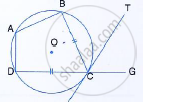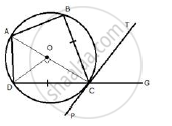Share

Abcd is a Cyclic Quadrilateral with Bc = Cd. Tc is Tangent to the Circle at Point C and Dc is Produced to Point G. If ∠Bcg = 108° and O is the Centre of the Circle Find: (I) Angle Bct (Ii) Angle Doc - ICSE Class 10 - Mathematics

Question

In the figure, ABCD is a cyclic quadrilateral with BC = CD. TC is tangent to the circle at point C and DC is produced to point G. If ∠BCG = 108° and O is the centre of the circle, find:
(i) Angle BCT
(ii) angle DOCSolutionJoin OC, OD and AC
i)
∠BCG + ∠BCD = 180° (Linear pair)
⇒ 180 °+  ∠BCD  = 180°
⇒ ∠BCD = 180°  -180°  =72°
BC = CD
∴ ∠DCP = ∠BCT
But, ∠BCT + ∠BCD + ∠DCP = 180°
∴ ∠BCT  + ∠BCT + 72° = 180°
2∠BCT = 180°  - 72°

∠BCT = 54°

ii)
PCT is a tangent and CA is a chord.
∠CAD  = ∠BCT = 54°
But arc DC subtends ∠DOC at the centre and ∠CAD at the
remaining part of the circle.
∴ ∠DOC = 2∠CAD = 2 × 54° = 108°

Is there an error in this question or solution?

APPEARS IN

Solution Abcd is a Cyclic Quadrilateral with Bc = Cd. Tc is Tangent to the Circle at Point C and Dc is Produced to Point G. If ∠Bcg = 108° and O is the Centre of the Circle Find: (I) Angle Bct (Ii) Angle Doc Concept: Cyclic Properties.
S